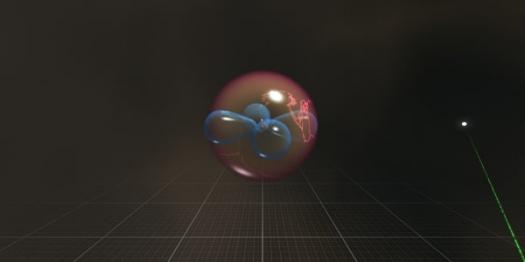# What's Up With Atomic Orbital?

10 Questions | Total Attempts: 120SettingsAtomic orbital represent the place around nucleus of an atom. Each Atomic orbital can sustain a certain amount of electrons. Complete this quiz to see how big is your knowledge in this field of study.

Related Topics
• 1.
What is the shape of a p orbital?
• A.

Dumbbell

• B.

Pyramidal

• C.

Sphere

• D.

It's just too complex to think about it

• 2.
What is the shape of an s orbital?
• A.

Double dumbbell

• B.

Sphere

• C.

Too complex to know

• D.

Dumbbell

• 3.
Which orbital has the least amount of energy?
• A.

All have the same amount of energy

• B.

P orbital

• C.

S orbital

• D.

D orbital

• 4.
How many electrons can the first energy level hold?
• A.

1

• B.

0

• C.

8

• D.

2

• 5.
How many electrons can each p orbital hold?
• A.

2

• B.

6

• C.

3

• D.

4

• 6.
How many d orbitals are there in a given sublevel?
• A.

3

• B.

5

• C.

1

• D.

7

• 7.
What is the highest occupied energy level?
• A.

1

• B.

2

• C.

3

• D.

4

• 8.
Which element has an Atomic number of 30?
• A.

Silver

• B.

Gold

• C.

Carbon

• D.

Zinc

• 9.
Which element has an Atomic number of 73?
• A.

Germanium

• B.

Gold

• C.

Sulfur

• D.

Selenium

• 10.
How many protons does Argon have?
• A.

15

• B.

18

• C.

23

• D.

26